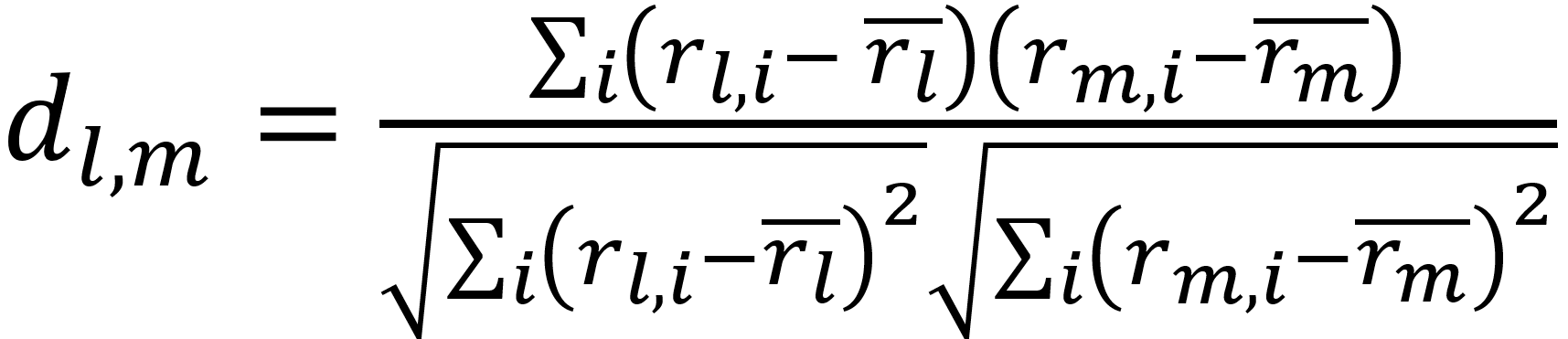# Memory Based

Earlier implementations of CF systems use the rating data to calculate the similarity between users or items and then make predictions or recommendations based on those similarities

There are two different types of memory-based CF algorithms, namely User Based and Item Based, which both use part or all of available user-item data to make predictions.

## User-based

• Assumes that similar items are usually liked by similar users, and then
• Identify those items to recommend them to new users.

## Item-based

• Both methods need to find the k-closest neighbors to the target.
• Usually databases have more users than items, and therefore user-based algorithms need to tackle the issue of dimensionality of the users.
• However, in the context of news, for example, the dimensionality of items becomes an issue to deal with rather than the users.

## Similarity Metrics

Pearson Correlation is one of the most common methods of calculating a similarity value between users. For example, the Pearson correlation dl,m between learners l and m measures how two variables (learners) linearly relate with each other and it's represented by a scale of -1 to +1 (zero indicates no correlation). The user-based Pearson Correlation similarity equation is as follows:Where the items i have been rated by learners l and m, and r̄l is the average rating of the co-rated items of the lth learner.

The details of the best neighbors learner-based algorithm are illustrated here
The memory model is also associated with a number of challenges